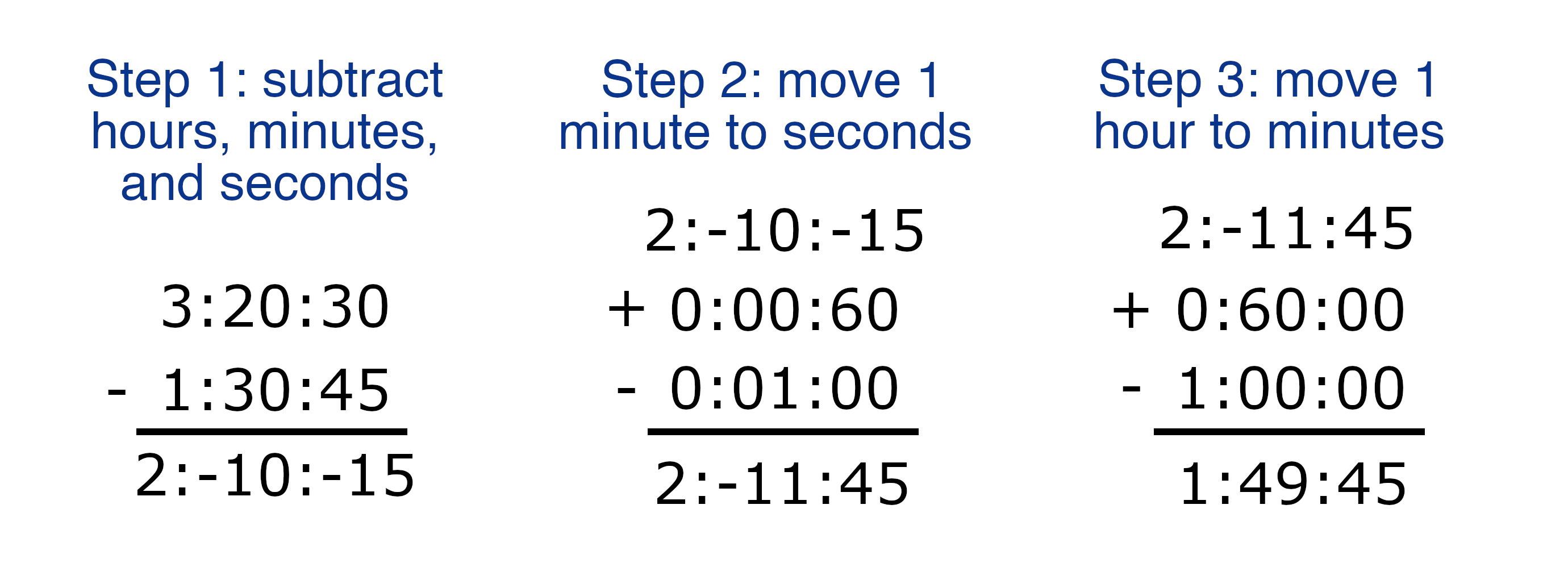# Subtract Times Calculator

To subtract one time from another, enter both times in hours, minutes, and seconds below.

Time One:
:
:
Time Two:
-
:
:

## How to Subtract One Time From Another

To subtract one time from another, follow a few simple steps.

Step One: subtract the second hours, minutes, and seconds from the first separately.

Step Two: if the seconds are a negative number, then add 60 seconds and subtract 1 minute.

Step Three: if the minutes are a negative number, then add 60 minutes and subtract 1 hour.

The result is the difference of the two numbers.

To convert the final time to total minutes or seconds, try our time to decimal calculator.

For example, subtract the time 1:30:45 from 3:20:30.

Subtract the hours, minutes, and seconds from the first time separately:
hours = 3 – 1 = 2 hours
minutes = 20 – 30 = -10 minutes
seconds = 30 – 45 = -15 seconds

Seconds are a negative number, so add 60 seconds and subtract 1 minute:
minutes = -10 – 1 = -11 minutes
seconds = -15 + 60 = 45 seconds

Minutes are a negative number, so add 60 minutes and subtract 1 hour:
hours = 2 – 1 = 1 hour
minutes = -11 + 60 = 49 minutes

Thus, the time difference is 1:49:45.